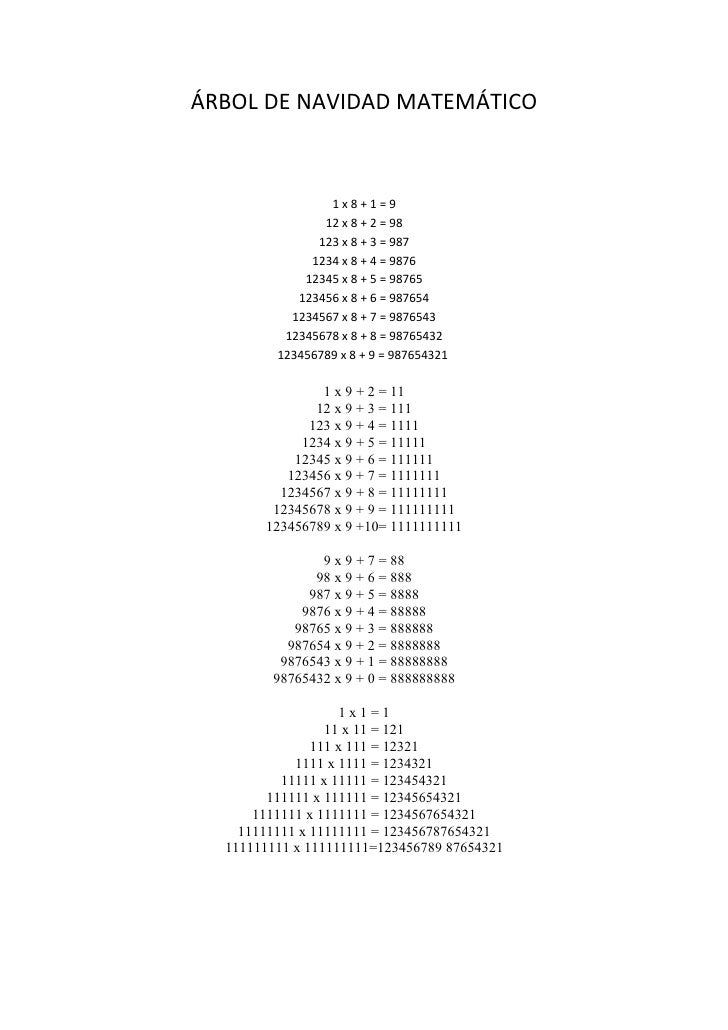Successfully reported this slideshow.
We use your LinkedIn profile and activity data to personalize ads and to show you more relevant ads. You can change your ad preferences anytime.Upcoming SlideShare
×

# ÁRBOL DE NAVIDAD MATEMÁTICO

1,094 views

Published on

Árbol de Navidad de la página web de la Esquizopedia

• Full Name
Comment goes here.

Are you sure you want to Yes No
Your message goes here• Be the first to comment

• Be the first to like this

### ÁRBOL DE NAVIDAD MATEMÁTICO

1. 1. ÁRBOL DE NAVIDAD MATEMÁTICO 1x8+1=9 12 x 8 + 2 = 98 123 x 8 + 3 = 987 1234 x 8 + 4 = 9876 12345 x 8 + 5 = 98765 123456 x 8 + 6 = 987654 1234567 x 8 + 7 = 9876543 12345678 x 8 + 8 = 98765432 123456789 x 8 + 9 = 987654321 1 x 9 + 2 = 11 12 x 9 + 3 = 111 123 x 9 + 4 = 1111 1234 x 9 + 5 = 11111 12345 x 9 + 6 = 111111 123456 x 9 + 7 = 1111111 1234567 x 9 + 8 = 11111111 12345678 x 9 + 9 = 111111111 123456789 x 9 +10= 1111111111 9 x 9 + 7 = 88 98 x 9 + 6 = 888 987 x 9 + 5 = 8888 9876 x 9 + 4 = 88888 98765 x 9 + 3 = 888888 987654 x 9 + 2 = 8888888 9876543 x 9 + 1 = 88888888 98765432 x 9 + 0 = 888888888 1x1=1 11 x 11 = 121 111 x 111 = 12321 1111 x 1111 = 1234321 11111 x 11111 = 123454321 111111 x 111111 = 12345654321 1111111 x 1111111 = 1234567654321 11111111 x 11111111 = 123456787654321 111111111 x 111111111=123456789 87654321